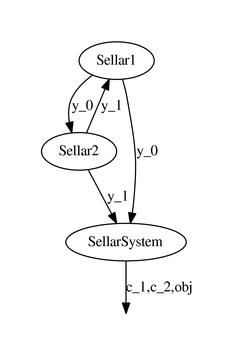# Coupling visualization¶

First of all, we need to instantiate the different MDODiscipline, e.g.

from gemseo.api import create_discipline

disciplines = create_discipline(['Sellar1', 'Sellar2', 'SellarSystem'])


## N2 chart¶

Then, we can represent the coupling structure of these disciplines by means of a N2 chart, also referred to as N2 diagram, N-squared diagram or N-squared chart.

### Description¶

For that, we consider the generate_n2_plot() API function:

from gemseo.api import generate_n2_plot

generate_n2_plot(disciplines)


and obtains the following N2 chart where:

• disciplines are on the diagonal,

• the input coupling variables of a discipline are located at the vertical of the discipline,

• the output coupling variables of a discipline are located at the horizontal of the discipline.

In this case, Sellar1 returns the coupling variable y_0 which is an input for Sellar2 and SellarSystem. Similarly, Sellar2 returns the coupling variable y_1 which is an input for Sellar1 and SellarSystem.

### Options¶

The first argument of the generate_n2_plot() API function is the list of MDODiscipline. This argument is mandatory while the others are optional:

• file_path: file path of the figure (default value: 'n2.pdf')

• show_data_names: if true, the names of the coupling data is shown otherwise, circles are drawn, which sizes depend on the number of coupling names (default value: True)

• save: if True, saved the figure to file_path (default value: True)

• show: if True, shows the plot (default value: False)

• figsize: tuple, size of the figure (default value: (15,10))

Here, when show_data_names is False, we obtain:

## Coupling graph¶

We can also represent this relation by means of a coupling graph.

### Description¶

For that, we consider the generate_coupling_graph() API function:

from gemseo.api import generate_coupling_graph

generate_coupling_graph(disciplines)


and obtains the following coupling graph where:

• disciplines are represented by bubbles,

• coupling variable flows are represented by arrows between disciplines,

• constraint and objectives functions are represented.

In this case, the coupling variable y_0 goes from Sellar1 to Sellar2 and from Sellar1 to SellarSystem. Similarly, the coupling variable y_1 goes from Sellar2 to Sellar1 and from Sellar2 to SellarSystem. Moreover, SellarSystem returns the value of the objective function and constraints.### Options¶

The first argument of the generate_coupling_graph() API function is the list of MDODiscipline. This argument is mandatory while the other is optional:

• file_path: file path of the figure (default value: 'coupling_graph.pdf')